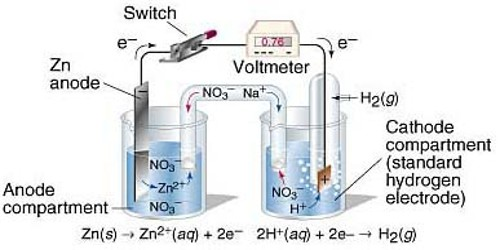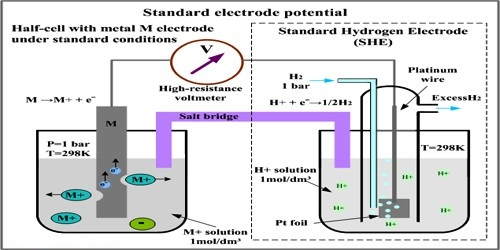Chemistry

# Determination of Standard Electrode Potential (SEP)Determination of Standard Electrode Potential (SEP)

For measuring the standard potential of an electrode (which is referred to as test electrode) the test electrode is combined with a standard hydrogen electrode (SHE) to form a cell as shown in Figure.

In the standard electrode the concentration of the ion has to be 1.0 mol L-1 and measurements have to be made at 250C. By convention the standard hydrogen electrode is placed on the left hand side and the test electrode on the right hand side so that the potential of the cell is given by:

E0cell = E0test electrode – E0SHE

But, E0SHE = 0.00. Hence, E0cell = E0test electrodeFigure: A test electrode is coupled with a standard hydrogen electrode

In other, a word with this experimental set up E0cell as measured is the standard potential of the test electrode. The potential of the cell is measured with the help of a high resistance voltmeter or a digital voltmeter.

E0cell is positive if SHE is the anode, i.e., electrons flow from the left side to the right side through the external circuit and E0test electrode is also positive. If, however, E0cell is negative then electrons flow from right side to the left, side, i.e., SHE acts as the cathode and E0test electrode iis negative.

For example, when the standard Zn electrode is combined with SHE, the E0cell is negative. So E0Zn is negative. On the other hand, under similar condition E0Cu is positive.

It is to be emphasized that in order to determine the cell potential the reduction potential of both the electrodes need to be used.

Reduction potential is for reduction reaction, e.g.,

Zn2+ (aq) + 2e → Zn (s)

Oxidation potential is for oxidation reaction, e.g.,

Zn (s) → Zn2+ (aq) + 2e

For the same electrode system reduction and oxidation potentials are numerically equal but opposite in sign.